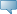# Lebanese American University WWW Information System

## Catalog Entries

Date
Sep 22, 2023Select the Course Number to get further detail on the course. Select the desired Schedule Type to find available classes for the course.

 MTH 304 - Differential Equations This course covers the topics of first order ordinary differential equations and applications, linear higher order differential equations and applications, systems of linear differential equations, series solutions of differential equations and solutions, and Laplace transforms. Course Learning Outcomes: 1) Students will be able to model and solve problems leading to first-order differential equations. 2) Students will be able to use symbolic methods to solve second order linear differential equations, and first order linear systems of differential equations. 3) Students will be able to compute Laplace transforms and inverse Laplace transforms, and to apply these to solve differential equations. 4) Students will be able to use direction fields and phase portraits to qualitatively analyze the solutions to differential equations. 3.000 Credit hours 3.000 Lecture hours Levels: Undergraduate Schedule Types: Lecture, Tutorial Computer Science & Mathematics Department